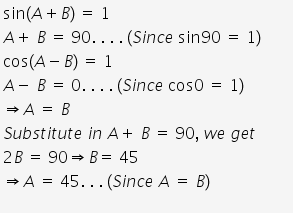# If sin(A+B) = 1 and cos(A-B) = 1 find A and B

If sin(A+B) = 1 and cos(A-B) = 1 find A and B.
EXPERTS, please explain the solution step by step.